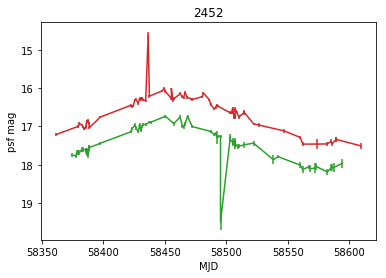## Light Curves of Minor Planets¶

This code finds named minor planets from ZTF that have many detections in the light curve, then makes plots of each light curve.

In :
import mysql.connector
%matplotlib inline
import matplotlib.pyplot as plt
import numpy as np


This SQL query finds the name of the minor planet (ssnamenr) and the number of corresponding detections (candidates), ordered with the biggest light curves first.

In :
import settings
msl = mysql.connector.connect(\
user    =settings.DB_USER, \
host    =settings.DB_HOST, \
database='ztf')
cursor = msl.cursor(buffered=True, dictionary=True)
query = 'SELECT ssnamenr, count(*) AS count FROM candidates '
query += 'GROUP BY ssnamenr ORDER BY count DESC LIMIT 20'
cursor.execute(query)
n = cursor.rowcount
print ('found %d minor planets' % n)

found 20 minor planets


For each of the light curves, split out the r and g filter detections (fid=0 and 1), and plot each one.

In :
nplot = 0
cursor2 = msl.cursor(buffered=True, dictionary=True)
for row in cursor:
count = row['count']
name  = row['ssnamenr']
if count > 200: continue  # fake values like 'null' and '-999' are very popular
print('Object %s has %d detections' % (name, count))
query = 'SELECT jd-2400000.5 AS mjd, magpsf, sigmapsf, fid FROM candidates WHERE ssnamenr = "%s"' % name
cursor2.execute(query)
magpsf   = [[],[]]
mjd      = [[],[]]
sigmapsf = [[],[]]
for row2 in cursor2:
fid = int(row2['fid'])-1
mjd     [fid].append(row2['mjd'])
magpsf  [fid].append(row2['magpsf'])
sigmapsf[fid].append(row2['sigmapsf'])
plt.errorbar(mjd, magpsf, yerr=sigmapsf, c="C2")
plt.errorbar(mjd, magpsf, yerr=sigmapsf, c="C3")
plt.xlabel("MJD")
plt.ylabel("psf mag")
plt.title("{}".format(name))
plt.gca().invert_yaxis()
plt.show()
nplot += 1
if nplot > 30: break

Object 3774 has 176 detections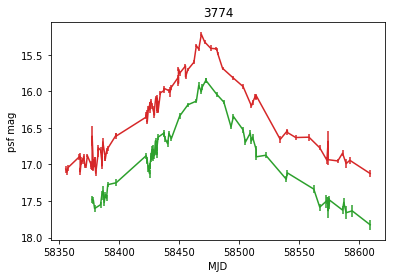Object 4759 has 168 detections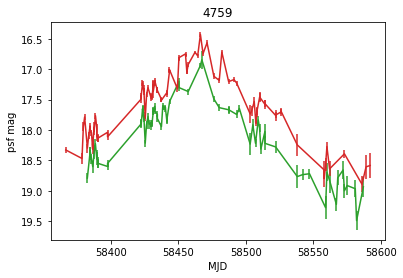Object 2231 has 165 detections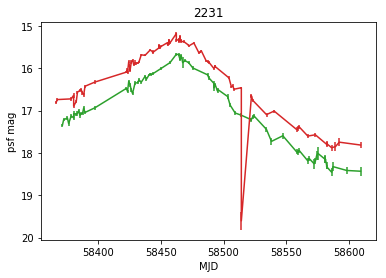Object 7623 has 164 detections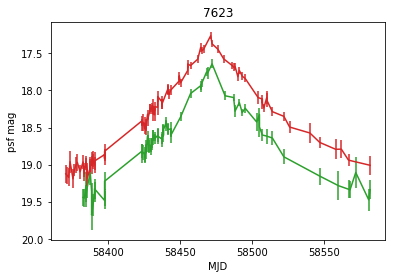Object 8127 has 155 detections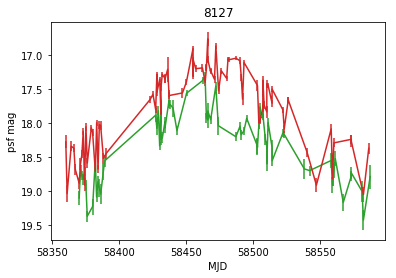Object 3397 has 153 detections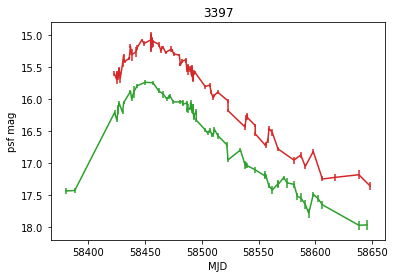Object 3852 has 153 detections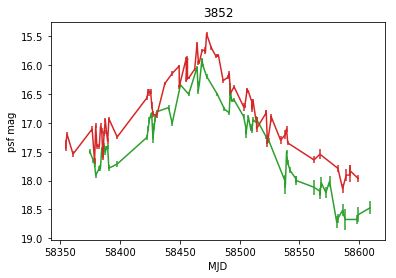Object 3471 has 153 detections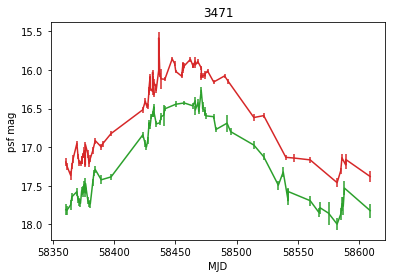Object 227 has 153 detections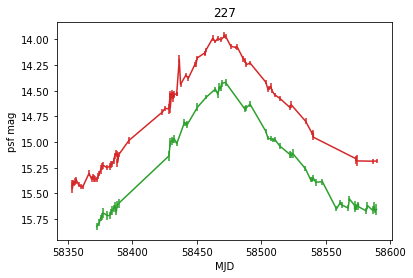Object 10330 has 152 detections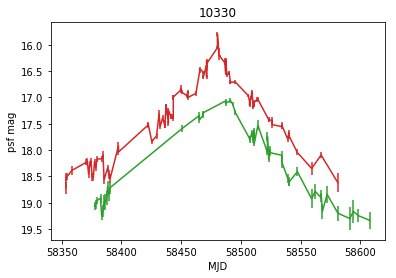Object 49640 has 151 detections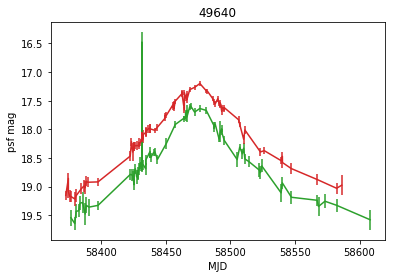Object 21228 has 150 detections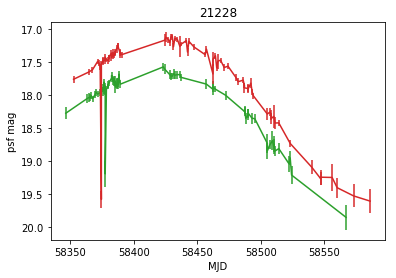Object 2865 has 150 detections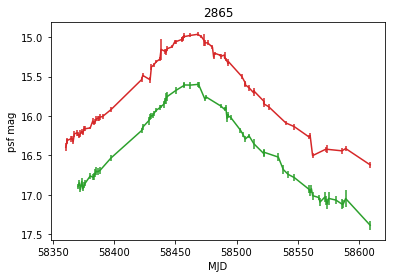Object 6522 has 150 detections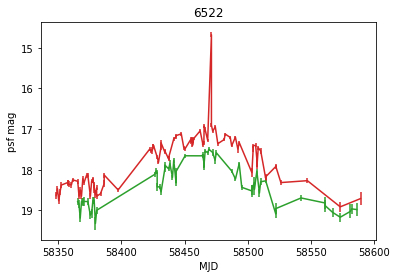Object 32259 has 149 detections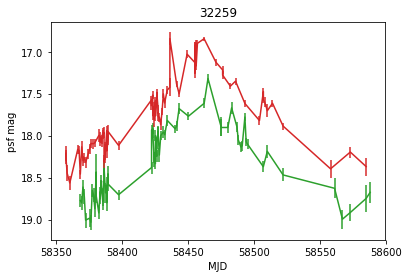Object 839 has 149 detections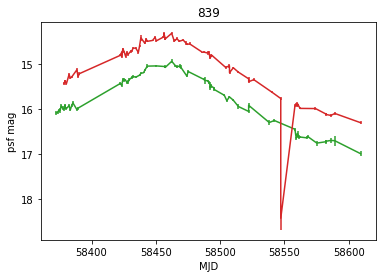Object 2544 has 148 detections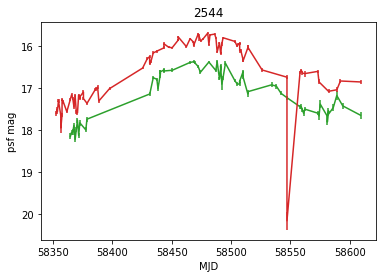Object 2452 has 147 detections